# SBI PO Reasoning Questions 2019 (Day-33) High Level-New Pattern

Dear Aspirants, the most awaited notification of SBI PO – 2019 has been released. We all know that new pattern questions are introducing every year in the SBI PO exam. Further, the questions are getting tougher and beyond the level of the candidate’s expectations.

Our IBPS Guide is providing High-Level New Pattern Reasoning Ability Questions for SBI PO 2019 so the aspirants can practice it on a daily basis. These questions are framed by our skilled experts after understanding your needs thoroughly. Aspirants can practice these high-level questions daily to familiarize with the exact exam pattern. We wish that your rigorous preparation leads you to a successful target of becoming SBI PO.

[WpProQuiz 5730]

### Click Here for SBI PO Pre 2019 High-Quality Mocks Exactly on SBI Standard

Directions (1–4): A string of letter is given as input. The further steps given are obtained by applying certain logic. Each step is a resultant of previous step only. Study the following information carefully and answer the questions given below it.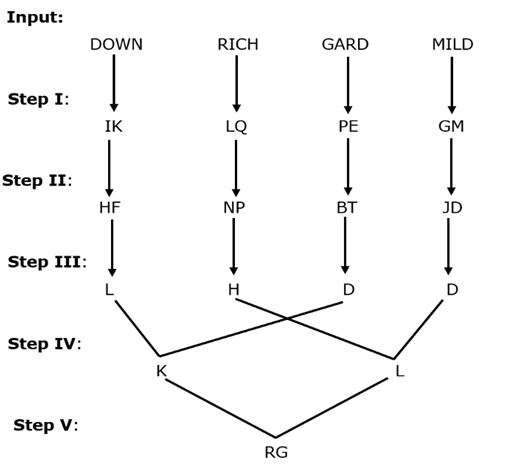As per the logic applied in above steps, find appropriate steps for given input: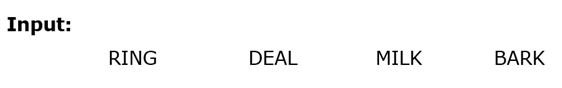1) If all the letters in step II are rearranged in reverse alphabetical order without any space from left to right then, which of the following letter appeared at third from right?

a) D

b) J

c) L

d) P

e) None of these

2) Which of the following string represents final output in step V?

a) RF

b) PG

c) KD

d) QH

e) None of these

3) If all the letters in step I are rearranged in alphabetical order without any space from left to right then, how many letters are there in English alphabetical series between 3rd letter and 7th letter from left?

a) Five

b) Three

c) Nine

d) Six

e) None of these

4) What is the difference of letter’s place value in the step IV?

a) 7

b) 2

c) 3

d) 9

e) None of these

Directions (5–7): In each question there are four statements numbered I to IV and followed by three conclusions numbered I to III. You have to take the given statement to be true even if they seem to be variance with commonly known facts. Read all the given statements and conclusions carefully and then decide for which set of statements all the conclusion logically follows.

5) Statements:

I. Some Car are Truck.

II. No Bus is Train.

III. P. No Bike is Car

IV. Q. Some Bus are Bike

Conclusions:

I. Some Bike are not Train.

II. Y.

III. Z.

a) P: Some Bike are Bus; Q: All Train are Car; Y: All Bike being Car is a Possibility; Z: Some Truck are not Bus.

b) P: Some Truck are Bike; Q: All Bike are Train; Y: Some Train are not Truck; Z: All Car being Train is a possibility.

c) P: Some Bike are Car; Q: All Bike are Bus; Y: Some Train are not Car; Z: Some Bus are not Truck.

d) P: No Bike is Car; Q: Some Bus are Bike; Y: Some Truck are not Bike; Z: Some Bus are not Car.

e) More than one option is true.

6) Statements:

I. No Pink is Red.

II. No Blue is Green.

III. P. Some Red are Blue

IV. Q. All Yellow are Red

Conclusions:

I. Some Yellow are not Pink.

II. Y.

III. Z.

a) P: All Blue are Yellow; Q: No Pink is Blue; Y: All Green being Yellow is a possibility; Z: Some Yellow are not Pink.

b) P: All Yellow are Red; Q: Some Yellow are Green; Y: All Red being Blue is a possibility; Z: Some Green are not Pink.

c) P: Some Green are Yellow; Q: Some Red are Yellow; Y: Some Yellow are not Blue; Z: All Red can never be Green.

d) P: Some Red are Blue; Q: All Yellow are Red; Y: Some Blue can never be Pink; Z: All Yellow being Red is a possibility.

e) More than one option is true.

7) Statements:

I. All Deer are Lion.

II. No Tiger is Horse.

III. P.

IV. Q.

Conclusions:

I. Some Wolf are not Horse.

II. Y.

III. Z.

a) P: Some Lion are Wolf; Q: No Horse is Wolf; Y: All Deer being Horse is a possibility; Z: Some Tiger are not Wolf.

b) P: All Wolf are Tiger; Q: Some Lion are Tiger; Y: All Deer can never be Horse; Z: Some Lion are not Horse.

c) P: No Lion is Horse; Q: Some Deer are Wolf; Y: All Deer being Horse is a possibility; Z: Some Deer are not Tiger.

d) P: Some Lion are Tiger; Q: Some Wolf are Tiger; Y: All Lion can never be Horse; Z: All Horse being Deer is a possibility.

e) More than one option is true.

Directions (8-10): Study the following information carefully and answer the questions given below:

HPEWU  BMKFD  SWQAH  DFTXI  JRCKI

8) If each consonant are replaced by previous letter and each vowel is replaced by its next letter, then which of the following word is second from the left end when all the words are arranged in ascending order?

a) BMKFD

b) SWQAH

c) HPEWU

d) DFTXI

e) None of these

9) If first and fifth letters are interchanged, second and fourth letters are interchanged within the word and third letter is replaced by its previous letter, then how many letter appear twice within the word?

a) None

b) One

c) Two

d) Three

e) None of these

10) If first and second letters are interchanged, third and fourth letters are replaced by its second next letter, then all the words are arranged in descending order, which of the following word is third from the right end?

a) SWQAH

b) HPEWU

c) JRCKI

d) DFTXI

e) None of these

Directions (1–4):

We have: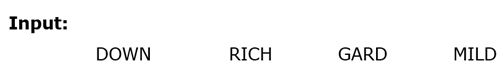Step I: In this step following logic is applied: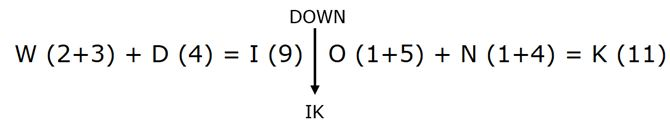Clearly, 1st letter in step I can be determined by sum of place value of 1st and 3rd letter. Similarly, 2nd letter in step I can be determined by sum of place value of 2nd and 4th letter.

Step II: In this step following logic is applied:

Clearly, result in the step II can be determined as follow: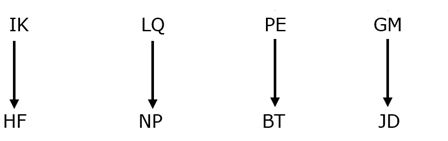• If place value of letter is even then, result can be determined by:

Letter + 4 = Result

L (12) + 4 = P (16)

• If place value of letter is odd then, result can be determined by:

Letter – 3 = Result

Q (17) – 3 = N (14)

Final result can be determined by interchanging letters in the result.

Thus, Final result of “LQ” àNP

Step III: In this step following logic is applied: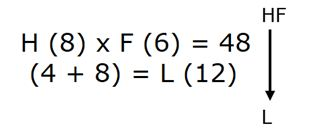Clearly, results in the step III can be determined as follows:

• Results can be determined by product of place value of letters.

H (8) x F (6) = 48

• Final result can be determined by sum of digits of result.

Result = (4 + 8) = L (12)

Step IV: In this step following logic is applied:

Clearly, results in the step V can be determined as follow: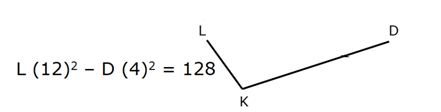• Result can be determined by difference square of place value of letters.

L (12)2 – D (4)2 = 128

• Final result can be determined by sum of digits obtained in the result.

(1 + 2 + 8) = K (11)

Step V: In this step following logic is applied: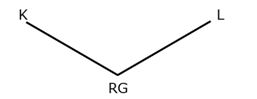• If place value of letter is odd then, result can be determined by:

Letter – 4 = Result

K (11) – 4 = G (7)

• If place value of letter is even then, result can be determined by:

Letter + 6 = Result

L (12) + 6 = R (18)

Final result can be determined by interchanging letters of the obtained two letters string.

Thus, Final result of the string “KL” –> RG

Thus, from above given steps we final following result for given input: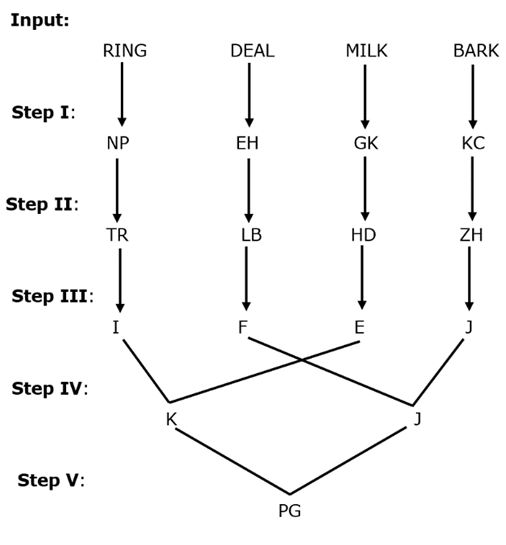After rearranging letters in reverse alphabetical order from left to right we have –> ZTRLHHDB

Clearly, third letter from right end = H

Hence, option E is correct choice.

Clearly, “PG” is the final output in the step V.

Hence, option B is correct choice.

After rearranging letters in alphabetical series we have àCEGHKKNP

Thus, letters between ‘G’ & ‘N’ in English alphabetical series = six.

Hence, option D is correct choice.

Clearly, letters in step III àK (11) and J (10)

Thus, required difference = (11 – 10) = 1

Hence, option E is correct choice.

From A we have: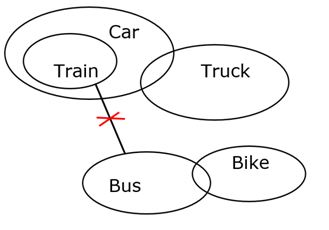Given conclusion: Some Bike are not Train. All Bike being Car is a Possibility. Some Truck are not Bus.

Clearly, there is no direct connection between Bus and Train, thus “Some Truck are not Bus” is not true.

Hence, option A is not correct.

From B we have: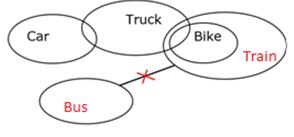Given conclusion: Some Bike are not Train. Some Train are not Truck.  All Car being Train is a possibility.

Clearly, there is no direct connection between Truck and Train, thus we can’t say “Some Train are not Truck”.

Hence, option B is not correct choice.

From C we have: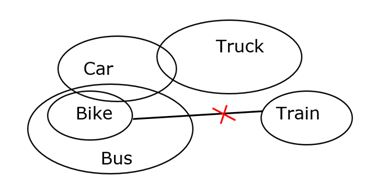Given conclusion: Some Bike are not Train. Some Train are not Car. Some Bus are not Truck.

Clearly, there is no direct connection between Train and Car, thus we can’t say “Some Train are not Car” is not true.

Hence, option C is not correct choice.

From D we have: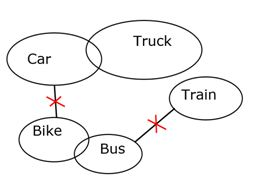Given conclusion: Some Bike are not Train. Some Truck are not Bike. Some Bus are not Car.

Clearly, all the above conclusion follows.

Hence, option D is correct choice.

From A we have: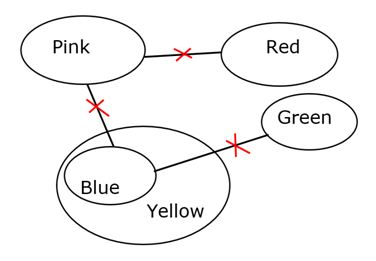Given conclusion: Some Yellow are not Pink. All Green being Yellow is a possibility.

Some Red are not Pink.

Clearly, all the above given conclusion are true.

Hence, option A is correct.

From B we have: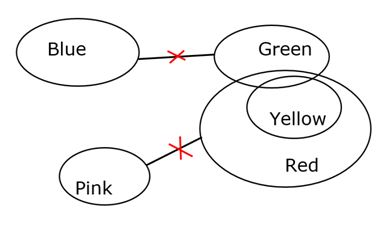Given conclusion: Some Yellow are not Pink. All Red being Blue is a possibility. Some Green are not Pink.

Clearly, Some Green are Red & No Green is Blue, thus “All Red can’t be Blue”.

Hence, option B is not correct choice.

From C we have: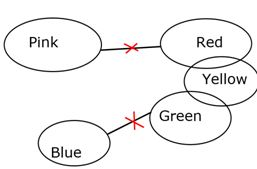Given conclusion: Some Yellow are not Pink. Some Yellow are not Blue. All Red can never be Green.

Clearly, there is no direct connection between Red and Green, thus “All Red being Green is a possibility”.

Hence, option C is not true.

From D we have: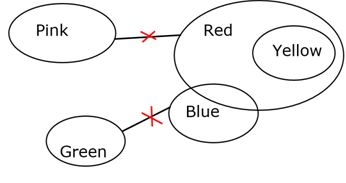Given Conclusion: Some Yellow are not Pink. Some Blue can never be Pink; Z: All Yellow being Red is a possibility.

Clearly, all the above given conclusion follows.

Hence, option D is correct.

Since, both option A and D are correct, thus option E is correct choice.

From A we have: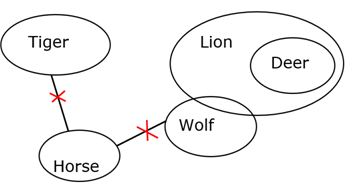Given conclusion: Some Wolf are not Horse. All Deer being Horse is a possibility. Some Tiger are not Wolf.

clearly, there is no direct connection between Tiger and Wolf, thus we can’t say “Some Tiger are not Wolf” is true.

Hence, option A is not true.

From B we have: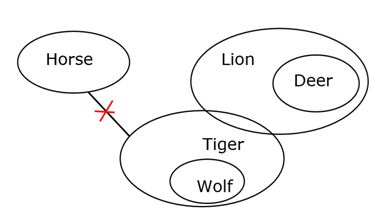Given conclusion: Some Wolf are not Horse. All Deer can never be Horse. Some Lion are not Horse.

Clearly, there is no direct connection between Deer and Horse, thus  “All Deer can be Horse”.

Hence, option B is not true.

From C we have: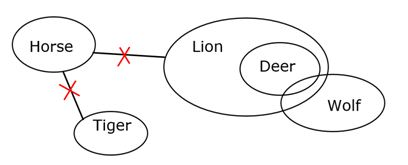Given conclusion: Some Wolf are not Horse. All Deer being Horse is a possibility; Z: Some Deer are not Tiger.

Clearly, no Horse is Lion and All Deer are Lion, thus we can say that “No Deer is Horse”. Then “All Deer being Horse is a Possibility” is not true.

Hence, option C is not correct choice.

From D we have: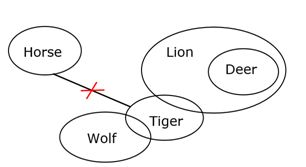Given conclusion: Some Wolf are not Horse. All Lion can never be Horse; All Horse being Deer is a possibility.

Clearly, all the above conclusion follows.

Hence, option D is correct choice.

Directions (8-10):

HPEWU  BMKFD  SWQAH  DFTXI  JRCKI

GOFVV   ALJEC   RVPBG   CESWJ  IQBJJ

ALJEC   CESWJ   GOFVV   IQBJJ  RVPBG

HPEWU  BMKFD  SWQAH  DFTXI  JRCKI

UWDPH  DFJMB   HAPWS  IXSFD  IKBRJ